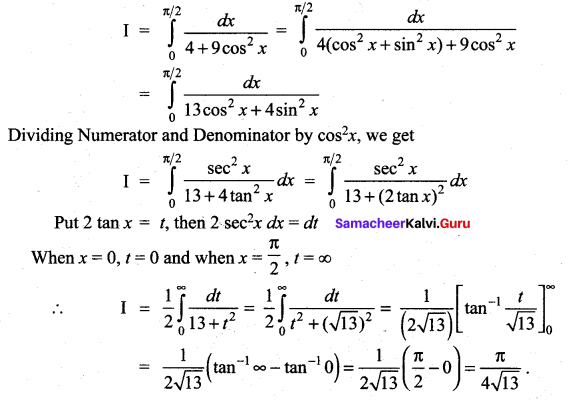## Tamilnadu Samacheer Kalvi 12th Maths Solutions Chapter 9 Applications of Integration Ex 9.5

Question 1.
Evaluate the following: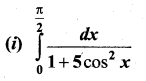Solution: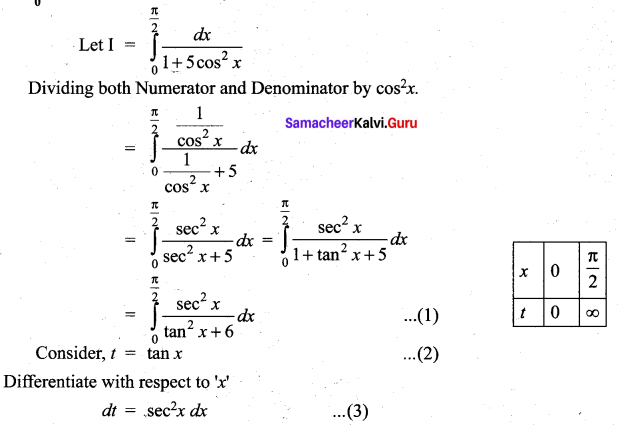Substitute (2) and (3) in (1), we get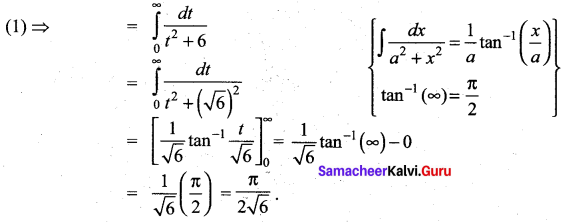(ii)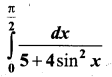Solution: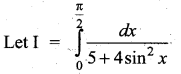Dividing both Numerator and Denominator by cos2 x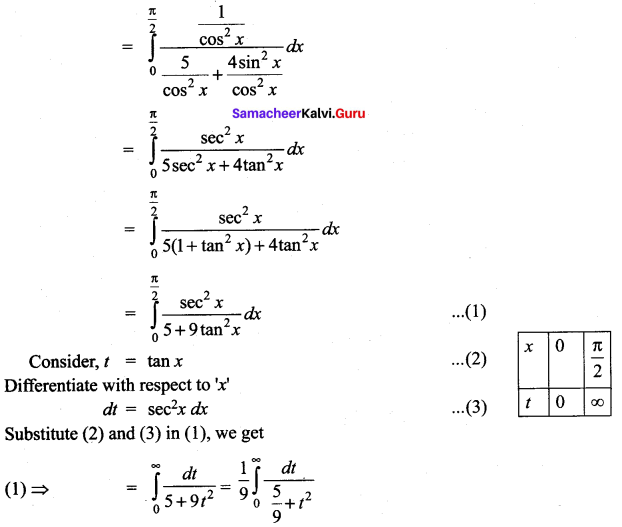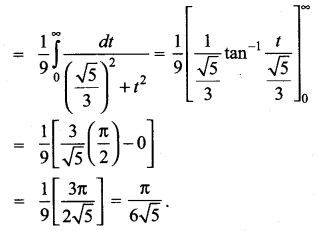### Samacheer Kalvi 12th Maths Solutions Chapter 9 Applications of Integration Ex 9.5 Additional Problems

Question 1.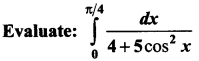Solution: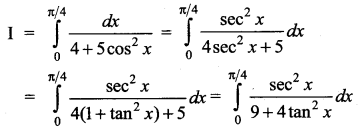Put 2 tan x, ∴ 2sc2 x dx = dt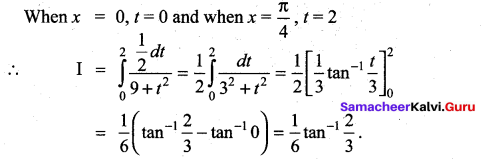Question 2.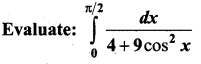Solution: